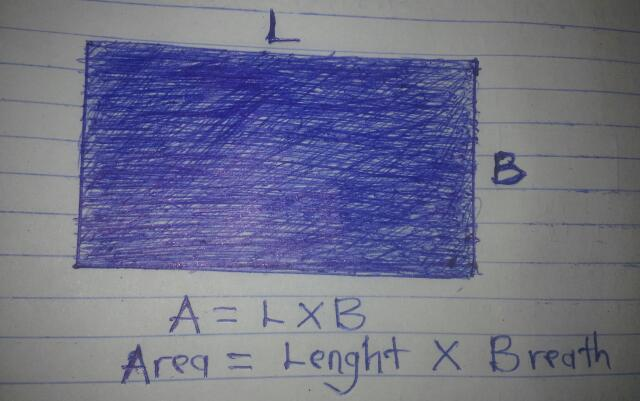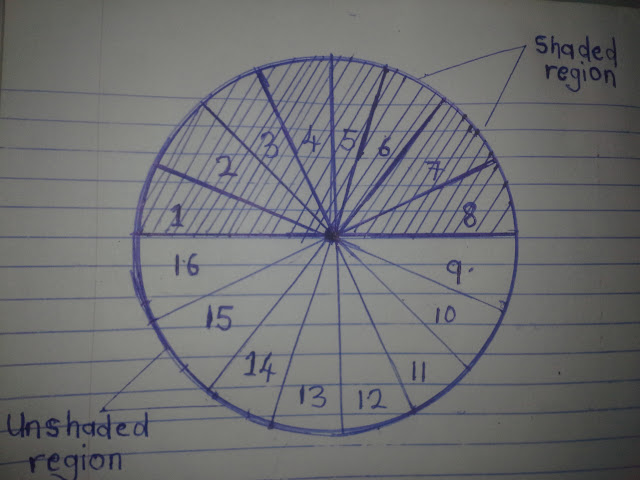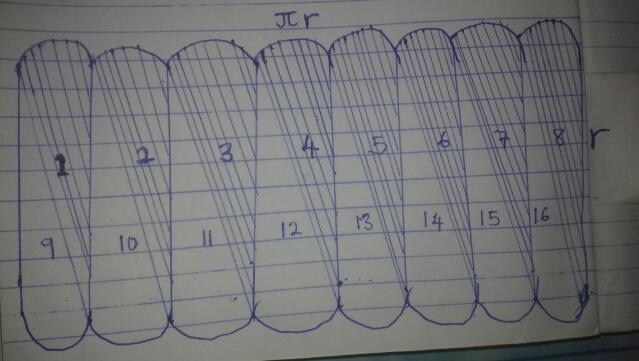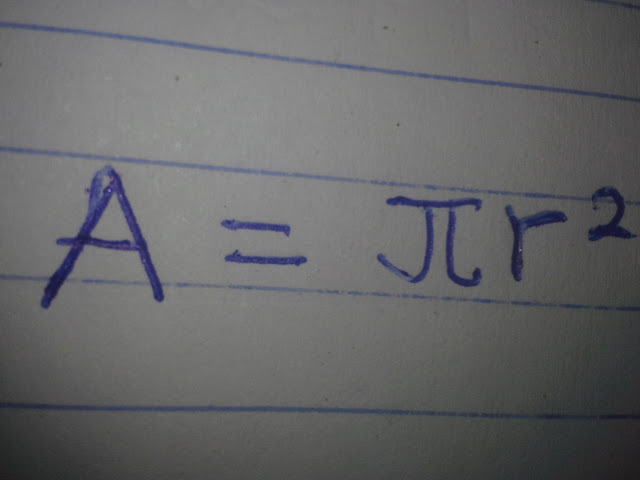# Teaching Your Child How To Find The Area Of A Circle.

Teaching And Learning Materials To Use For The Teaching
1) Circular Cut-out
3) Knife
4) Paint

INTRODUCTION
Make sure the child knows how to find the area of a rectangle.ACTIVITIES
Activity 1
Guide the child to measure the circumference of the circular cut-out.
Definition of Circumference: circumference of a circle is the distance around a circle.

Diameter(d) = 2r where r is the radius.

Activity 2
Let the child devide the circumference by the diameter to get pi( π ). That's, π=C/d

Activity 3
Discuss the ratio π=C/d with the child.

Activity 4
Guide the child to write this as C = πd = 2πr

Activity 5
Guide the child to draw on a piece of paper a circle with radius say 4cm.

Activity 6
Guide the child to cut and devide it into sixteen(16) equal parts. Number the parts from one(1) to sixteen(16)Activity 7
Assist the child to establish the fact that the length of the curved of the semi-circle is 2πr/2 = πr

Activity 8
Guide the child to arrange the Coloured and non-coloured sectors to form a rectangle.Activity 9
The child finds an approximate expression for length as πr and approximate expression for the breath as r

Activity 10
Guide the child to apply the formula of the area of a rectangle as A=LxB (where L is lenght and Breath or the Width) and relate this to the area of a circle. A = πr x r

Activity 11
The child simplifies this to getCONCLUSION
Let the child repeat the activity without your interference.